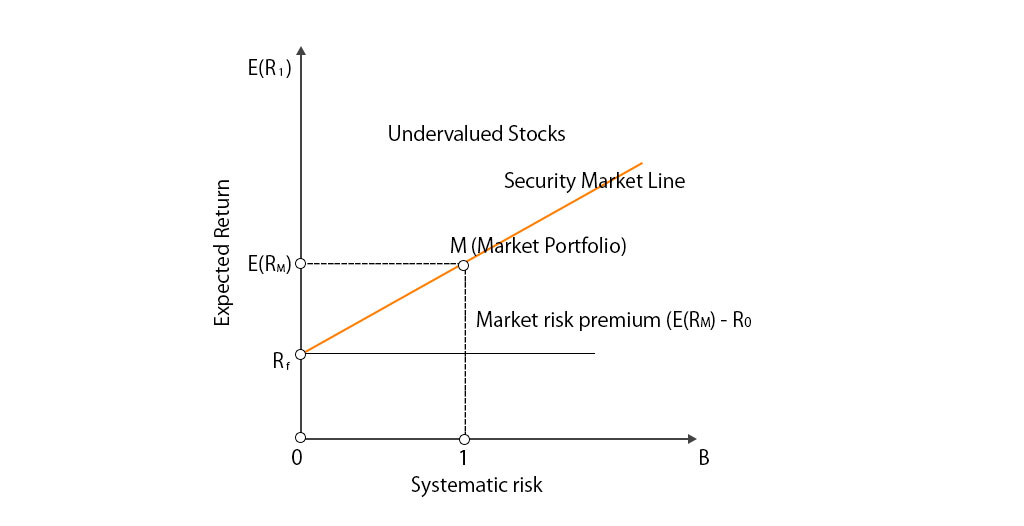# Securities Market Line

Security market line (SML) is the graphical representation of the Capital Asset Pricing Model (CAPM). SML gives the expected return of the market at different levels of systematic or market risk. It is also called ‘characteristic line’ where the x-axis represents beta or the risk of the assets and y-axis represents the expected return.

SML: E(Ri) = Rf + βi [E(RM) – Rf]

In the above security market line formula:

• E(Ri) is the expected return on the security
• Rf is the risk-free rate and represents the y-intercept of the SML
• βi is a non-diversifiable or systematic risk. It is the most important factor of SML. We will discuss in detail in this article.
• E(RM) is expected return on market portfolio M.
• E(RM) – Rf  is known as Market Risk Premium

The above security market line equation can be graphically represented as below:### Characteristics of Security Market Line (SML) are as below

• SML is a good representation of investment opportunity cost which provides the combination of risk-free asset and the market portfolio.
• Zero-beta security or zero-beta portfolio has an expected return on the portfolio which is equal to the risk-free rate
• The slope of the SML is determined by market risk premium which is: (E(RM) – Rf). Higher the market risk premium steeper the slope and vice-versa
• All the assets which are correctly priced are represented on Security Market Line (SML).
• The assets which are above the SML are undervalued as they give the higher expected return for a given amount of risk.
• The assets which are below the SML are overvalued as they have lower expected returns for the same amount of risk.

## One thought on “Securities Market Line”

error: Content is protected !!